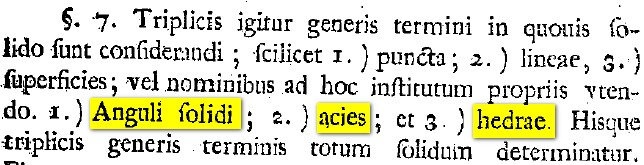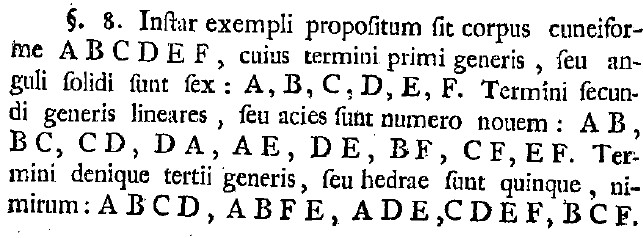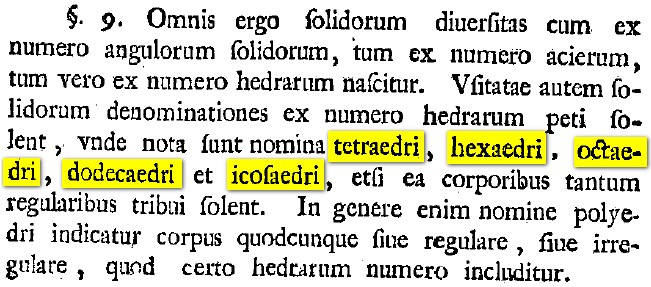# Investigating Euler's Polyhedral Formula Using Original Sources - Definitions and Examples

Author(s):

Now let us examine the content of the paper.

Euler spends the first six paragraphs motivating his topic; he wishes to put solid geometry on the same foundation as plane geometry. This section of Euler's paper is possibly the most difficult to translate in terms of vocabulary and grammar, and thus we will skip ahead to the mathematics.

This paper is full of results and examples, each of which is divided into a digestible length: paragraphs of only a few sentences. Once the reader is able to capture the mathematical content in a given paragraph (usually one fact per paragraph), the next paragraph may be explored. Here, we will refer to the paragraphs by number and highlight some of those which are especially suitable for use in the classroom.

In paragraph 7 (Figure 1), Euler mentions that there are three parts of the solid that will be considered: points, lines, and surfaces. The names he gives them, respectively, are:

• Anguli solidi (solid angles), the number of which Euler denotes by S; in today's notation, we call them vertices and denote this quantity by V.
• Acies (sharp edges), the number of which Euler denotes by A; we call them edges and use E.
• Hedrae (as in polyhedrae), the number of which Euler denotes by H; we call them faces and use F.Figure 1. V, E, F defined.

Euler also repeatedly refers to two additional quantities. The first of these is anguli planius, the plane angles of the polygons that constitute the faces of a given polyhedron. For example, a square consists of four plane angles; at each vertex of a cube, three squares meet. Therefore, since a cube has eight vertices, it contains a total of of twenty-four plane angles. The second quantity that appears is laternum, or sides of polygons. Using the example of a cube again: a cube has six faces that are squares, each of which has four sides. Therefore we would say that a cube has twenty-four laternum; this is different from the number of edges, which is twelve. As the reader has possibly discovered, every edge is the intersection of two polygonal faces along a single side, and thus in general, the number of edges is half the total number of sides of all faces. This fact is actually Euler's first Proposition, and will be discussed in detail later in our paper. Euler never assigns letters to represent the number of anguli planius or number of laternum, so to facilitate classroom discussion, you may want to declare that these quantities be denoted by P and L. Another early observation of Euler is that P = L, a fact that he will use repeatedly in his subsequent propositions.

Since these terms are new to Euler's audience (as well as our students), the pedagogically sound next step is to look at an example. Therefore, in paragraph 8, Euler discusses a cuneiforme, or wedge-shape. (The word cuneiforme also refers to the wedge-shaped style of writing used by the ancient Sumerians and Babylonians.) Euler includes a picture of a wedge, labels the vertices, and explicitly lists all six vertices, nine edges, and five faces.Figure 2. Euler's wedge-shaped example.

In paragraph 9, Euler mentions the five regular polyhedra, which we commonly call the Platonic solids today. (Note that the "hexahedron" refers to the cube.)Figure 3. Regular polyhedra are mentioned.

In paragraph 10, Euler proposes a general naming system for polyhedra based on the number of vertices and faces, not dissimilar from the genus-species system used to classify living organisms today. True to his style, after discussing generalities, Euler proceeds to give specific examples of the names of some common solids under his new system in paragraph 11. In particular, the wedge-shape previously considered shall be called a pentaedrum hexagonum, as it has five faces and six vertices. The other examples of solids mentioned by Euler are a triangular pyramid, a triangular prism, and a parallelepiped. It might aid student comprehension to review the Greek names for the numbers from one to twenty at this point.

These three sections naturally lend themselves to a classroom discussion. What would Euler call a pentagonal prism? What would a hexagonum octaedrum be called today? Students should be given some time to become acquainted with the geometric terms before moving on; Euler's presentation style is very conducive to this. Depending on the depth to which a teacher wishes to cover this topic, there are deeper questions that can be explored at this junction. What are the advantages and disadvantages to Euler's naming system? Is it possible that two different solids would have the same name under this system? What do we mean by "different" in this sense? For example, should we consider a cube and a truncated square pyramid as "different"?

Lee Stemkoski (Adelphi University), "Investigating Euler's Polyhedral Formula Using Original Sources - Definitions and Examples," Convergence (April 2010), DOI:10.4169/loci003297

## Dummy View - NOT TO BE DELETED

••••